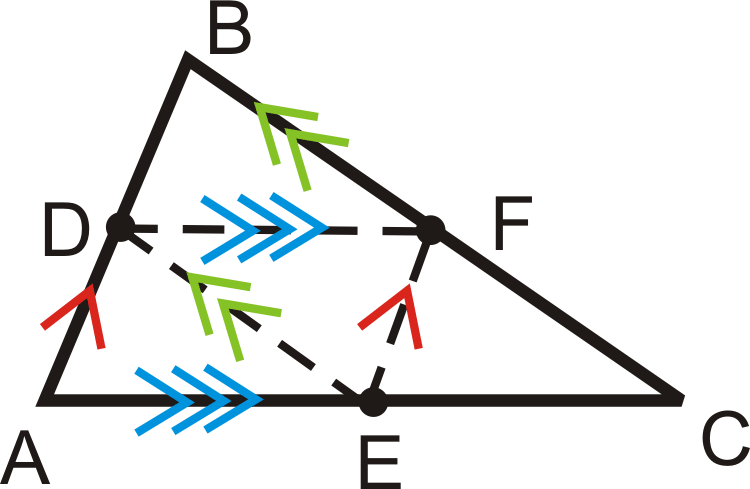# 4.19: Midsegment Theorem

•• Contributed by CK12
• CK12

Midsegment of a triangle joins the midpoints of two sides and is half the length of the side it is parallel to.

A line segment that connects two midpoints of the sides of a triangle is called a midsegment. $$\overline{DF}$$ is the midsegment between $$\overline{AB}$$ and $$\overline{BC}$$.Figure $$\PageIndex{1}$$

The tic marks show that $$D$$ and $$F$$ are midpoints. $$\overline{AD}\cong \overline{DB}$$ and $$\overline{BF}\cong \overline{FC}$$. For every triangle there are three midsegments.Figure $$\PageIndex{2}$$

There are two important properties of midsegments that combine to make the Midsegment Theorem. The Midsegment Theorem states that the midsegment connecting the midpoints of two sides of a triangle is parallel to the third side of the triangle, and the length of this midsegment is half the length of the third side. So, if $$\overline{DF}$$ is a midsegment of $$\Delta ABC$$, then $$DF=\dfrac{1}{2}AC=AE=EC$$ and $$\overline{DF} \parallel \overline{AC}$$.Figure $$\PageIndex{3}$$

Note that there are two important ideas here. One is that the midsegment is parallel to a side of the triangle. The other is that the midsegment is always half the length of this side.

What if you were given $$\Delta FGH$$ and told that $$\overline{JK}$$ was its midsegment? How could you find the length of $$JK$$ given the length of the triangle's third side, $$FH$$?

Example $$\PageIndex{1}$$

Find the value of $$x$$ and AB. $$A$$ and $$B$$ are midpoints.Figure $$\PageIndex{4}$$

Solution

$$AB=34\div 2=17$$. To find $$x$$, set $$3x−1$$ equal to 17.

\begin{align*} 3x−1&=17 \\ 3x&=18 \\ x&=6\end{align*}

Example $$\PageIndex{2}$$

True or false: If a line passes through two sides of a triangle and is parallel to the third side, then it is a midsegment.

Solution

This statement is false. A line that passes through two sides of a triangle is only a midsegment if it passes through the midpoints of the two sides of the triangle.

Example $$\PageIndex{3}$$

The vertices of $$\Delta LMN$$ are $$L(4,5),\: M(−2,−7)\:and\: N(−8,3)$$. Find the midpoints of all three sides, label them O, P and Q. Then, graph the triangle, plot the midpoints and draw the midsegments.

Solution

To solve this problem, use the midpoint formula 3 times to find all the midpoints. Recall that the midpoint formula is $$\left(\dfrac{x_1+x_2}{2},\dfrac{y_1+y_2}{2}\right)$$.

$$L$$ and $$M=\left(\dfrac{4+(−2)}{2}, \dfrac{5+(−7)}{2}\right)=(1,−1),\: point\: O$$

$$M$$ and $$N=\left(\dfrac{−2+(−8)}{2},\dfrac{−7+3}{2}\right)=(−5,−2),\: point\: P$$

$$L$$ and $$N=\left(\dfrac{4+(−8)}{2}, \dfrac{5+3}{2}\right)=(−2,4),\: point\: Q$$Figure $$\PageIndex{5}$$

Example $$\PageIndex{4}$$Figure $$\PageIndex{6}$$

Mark all the congruent segments on $$\Delta ABC$$ with midpoints $$D$$, $$E$$, and $$F$$.

Solution

Drawing in all three midsegments, we have:Figure $$\PageIndex{7}$$

Also, this means the four smaller triangles are congruent by SSS.

Now, mark all the parallel lines on $$\Delta ABC$$, with midpoints $$D$$, $$E$$, and $$F$$.Figure $$\PageIndex{8}$$

Example $$\PageIndex{5}$$

$$M$$, $$N$$, and $$O$$ are the midpoints of the sides of $$\Delta \(x$$YZ\).Figure $$\PageIndex{9}$$

Solution

Find $$MN$$, $$XY$$, and the perimeter of $$\Delta \(x$$YZ\).

Use the Midsegment Theorem:

$$MN=OZ=5$$

$$XY=2(ON)=2\cdot 4=8$$

Add up the three sides of $$\Delta XYZ$$ to find the perimeter.

$$XY+YZ+XZ=2\cdot 4+2\cdot 3+2\cdot 5=8+6+10=24$$

Remember: No line segment over MN means length or distance.

## Review

Determine whether each statement is true or false.

1. The endpoints of a midsegment are midpoints.
2. A midsegment is parallel to the side of the triangle that it does not intersect.
3. There are three congruent triangles formed by the midsegments and sides of a triangle.
4. There are three midsegments in every triangle.

R, S, T, and U are midpoints of the sides of $$\Delta XPO$$ and $$\Delta YPO$$Figure $$\PageIndex{10}$$
1. If $$OP=12$$, find $$RS$$ and $$TU$$.
2. If $$RS=8$$, find $$TU$$.
3. If $$RS=2x$$, and $$OP=20$$, find $$x$$ and $$TU$$.
4. If $$OP=4x$$ and $$RS=6x−8$$, find $$x$$.

For questions 9-15, find the indicated variable(s). You may assume that all line segments within a triangle are midsegments.

1.Figure $$\PageIndex{11}$$
2.Figure $$\PageIndex{12}$$
3.Figure $$\PageIndex{13}$$
4.Figure $$\PageIndex{14}$$
5.Figure $$\PageIndex{15}$$
6.Figure $$\PageIndex{16}$$
7.Figure $$\PageIndex{17}$$
8. The sides of $$\Delta XYZ$$ are 26, 38, and 42. $$\Delta ABC$$ is formed by joining the midpoints of $$\Delta XYZ$$.
1. What are the lengths of the sides of $$\Delta ABC$$?
2. Find the perimeter of $$\Delta ABC$$.
3. Find the perimeter of $$\Delta XYZ$$.
4. What is the relationship between the perimeter of a triangle and the perimeter of the triangle formed by connecting its midpoints?

Coordinate Geometry Given the vertices of $$\Delta ABC$$ below find the midpoints of each side.

1. $$A(5,−2),\: B(9,4)\: and\: C(−3,8)$$
2. $$A(−10,1),\: B(4,11)\: and \:C(0,−7)$$
3. $$A(−1,3),\: B(5,7)\: and\: C(9,−5)$$
4. $$A(−4,−15),\: B(2,−1)\: and\: C(−20,11)$$

## Vocabulary

Term Definition
midsegment A midsegment connects the midpoints of two sides of a triangle or the non-parallel sides of a trapezoid.
Congruent Congruent figures are identical in size, shape and measure.
Midpoint Formula The midpoint formula says that for endpoints $$(x_1,y_1)$$ and $$(x_2,y_2)$$, the midpoint is (\dfrac{x_1+x_2}{2}, \frac{y_1+y_2}{2})\).